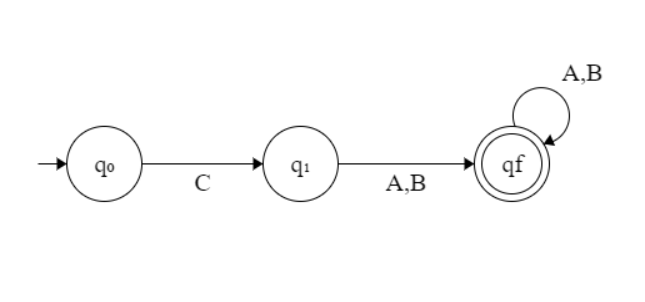Related Articles

# Program to construct DFA for Regular Expression C( A + B)+

• Last Updated : 24 May, 2021

Given a string S, the task is to design a Deterministic Finite Automata (DFA) for accepting the language L = C (A + B)+. If the given string is accepted by DFA, then print “Yes”. Otherwise, print “No”.

Examples:

Input: S = “CABABABAB”
Output: Yes
Explanation: The given string is of the form C(A + B)+ as the first character is C and it is followed by A or B.

Input: S = “ABAB”
Output: No

Approach: The idea is to interpret the given language L = C (A + B)+ and for the regular expression of the form C(A + B)+, the following is the DFA State Transition Diagram:Follow the steps below to solve the problem:

• If the given string is of length less than equal to 1, then print “No”.
• If the first character is always C, then traverse the remaining string and check if any of the characters is A or B.
• If there exists any character other than A or B while traversing in the above step, then print “No”.
• Otherwise, print “Yes”.

Below is the implementation of the above approach:

## C++

 `// C++ program for the above approach` `#include ``using` `namespace` `std;` `// Function to find whether the given``// string is Accepted by the DFA``void` `DFA(string str, ``int` `N)``{``    ``// If n <= 1, then print No``    ``if` `(N <= 1) {``        ``cout << ``"No"``;``        ``return``;``    ``}` `    ``// To count the matched characters``    ``int` `count = 0;` `    ``// Check if the first character is C``    ``if` `(str == ``'C'``) {``        ``count++;` `        ``// Traverse the rest of string``        ``for` `(``int` `i = 1; i < N; i++) {` `            ``// If character is A or B,``            ``// increment count by 1``            ``if` `(str[i] == ``'A'` `|| str[i] == ``'B'``)``                ``count++;``            ``else``                ``break``;``        ``}``    ``}``    ``else` `{` `        ``// If the first character``        ``// is not C, print -1``        ``cout << ``"No"``;``        ``return``;``    ``}` `    ``// If all characters matches``    ``if` `(count == N)``        ``cout << ``"Yes"``;``    ``else``        ``cout << ``"No"``;``}` `// Driver Code``int` `main()``{``    ``string str = ``"CAABBAAB"``;``    ``int` `N = str.size();``    ``DFA(str, N);` `    ``return` `0;``}`

## Java

 `// Java program for the above approach``import` `java.util.*;``class` `GFG``{` `  ``// Function to find whether the given``  ``// String is Accepted by the DFA``  ``static` `void` `DFA(String str, ``int` `N)``  ``{` `    ``// If n <= 1, then print No``    ``if` `(N <= ``1``)``    ``{``      ``System.out.print(``"No"``);``      ``return``;``    ``}` `    ``// To count the matched characters``    ``int` `count = ``0``;` `    ``// Check if the first character is C``    ``if` `(str.charAt(``0``) == ``'C'``)``    ``{``      ``count++;` `      ``// Traverse the rest of String``      ``for` `(``int` `i = ``1``; i < N; i++)``      ``{` `        ``// If character is A or B,``        ``// increment count by 1``        ``if` `(str.charAt(i) == ``'A'` `||``            ``str.charAt(i) == ``'B'``)``          ``count++;``        ``else``          ``break``;``      ``}``    ``}``    ``else``    ``{` `      ``// If the first character``      ``// is not C, print -1``      ``System.out.print(``"No"``);``      ``return``;``    ``}` `    ``// If all characters matches``    ``if` `(count == N)``      ``System.out.print(``"Yes"``);``    ``else``      ``System.out.print(``"No"``);``  ``}` `  ``// Driver Code``  ``public` `static` `void` `main(String[] args)``  ``{``    ``String str = ``"CAABBAAB"``;``    ``int` `N = str.length();``    ``DFA(str, N);``  ``}``}` `// This code is contributed by 29AjayKumar`

## Python3

 `# Python3 program for the above approach` `# Function to find whether the given``# is Accepted by the DFA``def` `DFA(``str``, N):``    ` `    ``# If n <= 1, then prNo``    ``if` `(N <``=` `1``):``        ``print``(``"No"``)``        ``return` `    ``# To count the matched characters``    ``count ``=` `0` `    ``# Check if the first character is C``    ``if` `(``str``[``0``] ``=``=` `'C'``):``        ``count ``+``=` `1` `        ``# Traverse the rest of string``        ``for` `i ``in` `range``(``1``, N):` `            ``# If character is A or B,``            ``# increment count by 1``            ``if` `(``str``[i] ``=``=` `'A'` `or` `str``[i] ``=``=` `'B'``):``                ``count ``+``=` `1``            ``else``:``                ``break``    ``else``:``        ``# If the first character``        ``# is not C, pr-1``        ``print``(``"No"``)``        ``return` `    ``# If all characters matches``    ``if` `(count ``=``=` `N):``        ``print``(``"Yes"``)``    ``else``:``        ``print``(``"No"``)` `# Driver Code``if` `__name__ ``=``=` `'__main__'``:``    ``str` `=` `"CAABBAAB"``    ``N ``=` `len``(``str``)``    ``DFA(``str``, N)` `# This code is contributed by mohit kumar 29.`

## C#

 `// C# program for the above approach``using` `System;``class` `GFG``{` `  ``// Function to find whether the given``  ``// String is Accepted by the DFA``  ``static` `void` `DFA(``string` `str, ``int` `N)``  ``{` `    ``// If n <= 1, then print No``    ``if` `(N <= 1)``    ``{``      ``Console.Write(``"No"``);``      ``return``;``    ``}` `    ``// To count the matched characters``    ``int` `count = 0;` `    ``// Check if the first character is C``    ``if` `(str == ``'C'``) {``      ``count++;` `      ``// Traverse the rest of String``      ``for` `(``int` `i = 1; i < N; i++) {` `        ``// If character is A or B,``        ``// increment count by 1``        ``if` `(str[i] == ``'A'``            ``|| str[i] == ``'B'``)``          ``count++;``        ``else``          ``break``;``      ``}``    ``}``    ``else` `{` `      ``// If the first character``      ``// is not C, print -1``      ``Console.Write(``"No"``);``      ``return``;``    ``}` `    ``// If all characters matches``    ``if` `(count == N)``      ``Console.Write(``"Yes"``);``    ``else``      ``Console.Write(``"No"``);``  ``}` `  ``// Driver Code``  ``static` `public` `void` `Main()``  ``{` `    ``string` `str = ``"CAABBAAB"``;``    ``int` `N = str.Length;``    ``DFA(str, N);``  ``}``}` `// This code is contributed by Dharanendra L V`

## Javascript

 ``
Output:
`Yes`

Time Complexity: O(N)
Auxiliary Space: O(1)

Attention reader! Don’t stop learning now. Get hold of all the important DSA concepts with the DSA Self Paced Course at a student-friendly price and become industry ready.  To complete your preparation from learning a language to DS Algo and many more,  please refer Complete Interview Preparation Course.

In case you wish to attend live classes with experts, please refer DSA Live Classes for Working Professionals and Competitive Programming Live for Students.

My Personal Notes arrow_drop_up